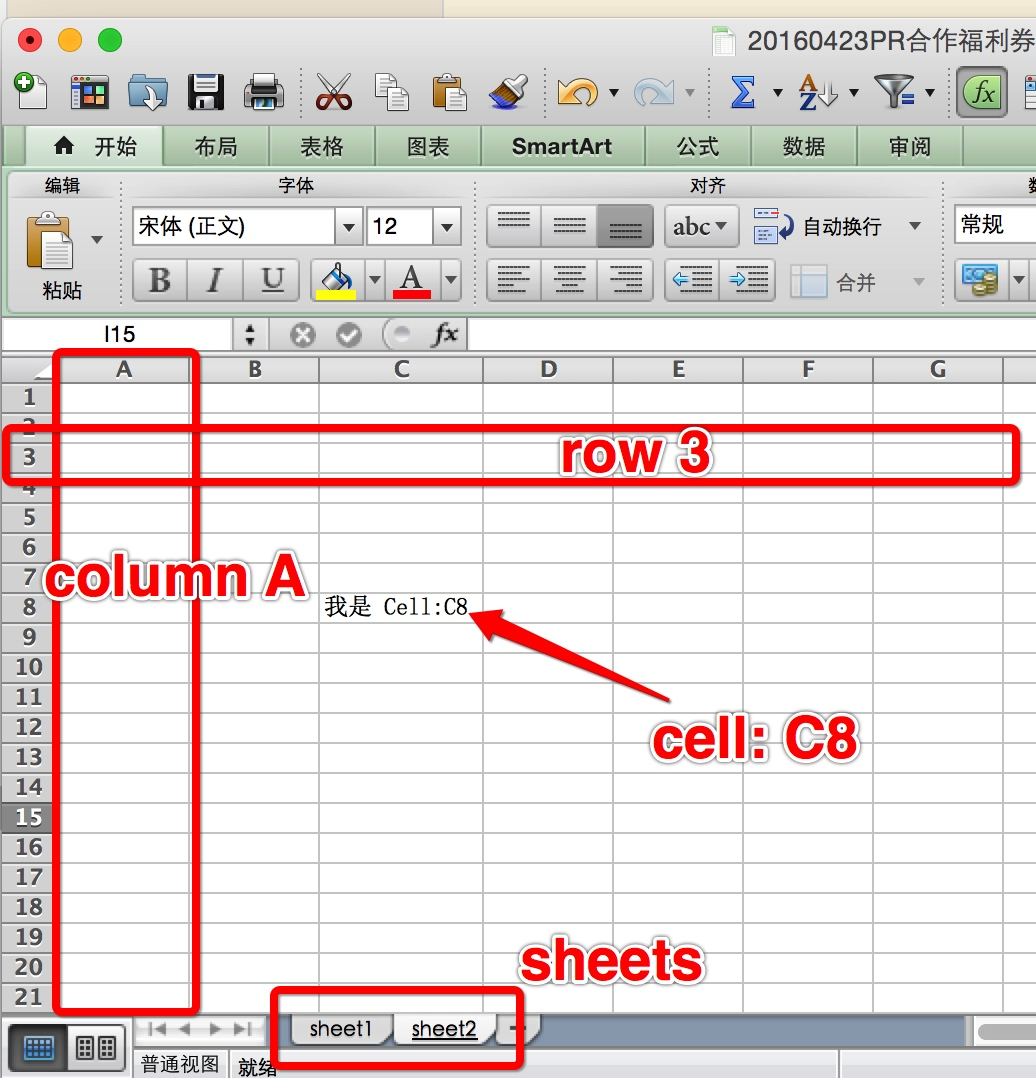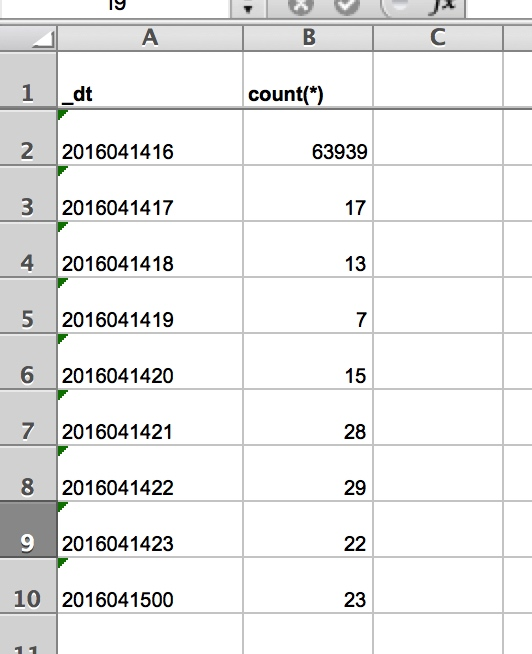python 读写 excel 有好多选择，但是，方便操作的库不多，在我尝试了几个库之后，我觉得两个比较方便的库分别是
xlrd/xlwt、openpyxl。之所以推荐两个库是因为这两个库分别操作的是不同版本的 excel，xlrd 操作的是 xls/xlxs 格式的 excel，而 openpyxl 只支持 xlxs 格式的 excel，openpyxl 使用起来会更方便一些，所以如果你只操作 xlxs 文件的话，那么可以优先选择 openpyxl，如果要兼容 xls 的话，那就用 xlrd/xlwt 吧。

updated at：2020-01-05 22:55

## excel 概念

workbook: 在各种库中，workbook 其实就是一个 excel 文件
sheet: 在一个 excel 文件中，可能会有多个 sheet，一个 sheet 可以看做一张表
row: row 其实就是一张表中的一行，正常是用数字 1、2、3、4 表示
column: column 就是一张表中的一列，正常用字母 A、B、C、D 表示
cell: cell 就是一张表中的一格，可以用 row + column 的组合来表示，例如： A3## xlrd/xlwt 读写 xls

1. 安装 xlrd 和 xlwt

xlrd 和 xlwt 都可以通过 pip 直接安装。

``    pip install xlrd``pip install xlwt``
1. 读取 excel``    import xlrd``    # 打开excel 文件``    data = xlrd.open_workbook('query_result.xls')``    # 通过名称获取工作表``    table = data.sheet_by_name(u'Tablib Dataset')``    # 获取表格的行数和列数``    nrows = table.nrows``    ncols = table.ncols``    for i in range(nrows):``        # 获取一行的数据，返回数组``        row_values = table.row_values(i)``            for i in range(ncols):``                print row_values[i]``

``# 用table直接获取单元格的值``cell_A1 = table.cell(0,0).value``cell_C4 = table.cell(2,3).value``

``# 使用行列索引来获取值``cell_A1 = table.row(0).value``cell_A2 = table.col(1).value``

3. 写出 excel

``import xlwt``# 创建工作簿``f = xlwt.Workbook() ``# 创建一个 user_info 的 sheet``sheet1 = f.add_sheet(u'user_info',cell_overwrite_ok=True)``rows = [['姓名', '性别', '年龄', '身高', '体重'],``          ['张三', '男', '18', '166', '60'],``          ['李四', '未知', '未知', '177', '88']]``for i in xrange(len(rows)):``    row = rows[i]``        for j in xrange(len(row)):``            # 以 cell 为单位写出单元格``            sheet1.write(i, j, rows[i][j])``# 别忘了保存``f.save('/Users/imaygou/Code/test/test.xls')``

## 一些问题

1. 读取excel中单元格内容为日期的方式

python读取excel中单元格的内容返回的有5种类型，即ctype:

``    ctype :  0 empty, 1 string, 2 number, 3 date, 4 boolean, 5 error``

``    >>> sheet2.cell(2,2).value  #1990/2/22``33656.0``

``    >>> xlrd.xldate_as_tuple(sheet2.cell_value(2,2),workbook.datemode)``(1992, 2, 22, 0, 0, 0)``

``    def convert_date(sheet, row, col):``    data = sheet.cell(row,col)``    if (data.ctype == 3):``        date_value = xlrd.xldate_as_tuple(sheet.cell_value(rows,3),book.datemode)``        data = date(*date_value[:3]).strftime('%Y/%m/%d')``    return data``
1. 读取合并单元格的内容
这个是真没技巧，只能获取合并单元格的第一个cell的行列索引，才能读到值，读错了就是空值。

2. 获取合并的单元格
读取文件的时候需要将formatting_info参数设置为True，默认是False，所以上面获取合并的单元格数组为空，

``    >>> workbook = xlrd.open_workbook(r'F:demo.xlsx',formatting_info=True)``>>> sheet2 = workbook.sheet_by_name('sheet2')``>>> sheet2.merged_cells``[(7, 8, 2, 5), (1, 3, 4, 5), (3, 6, 4, 5)]``

merged_cells返回的这四个参数的含义是：
(row,row_range,col,col_range),其中[row,row_range)包括row,不包括row_range,col也是一样，即(1,
3, 4, 5)的含义是：